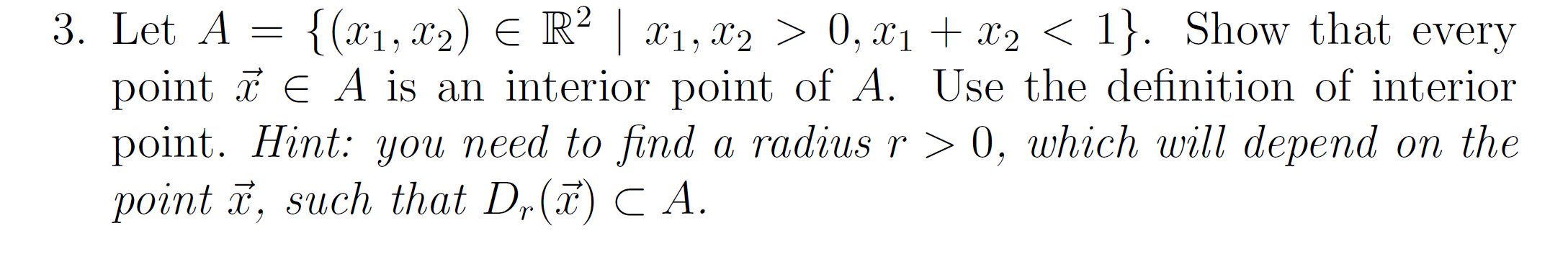# Question Solved1 AnswerThe set A is a triangle with dotted line which means it is a open set, and I need graph. 3. Let A = {(x1, x2) E R² | x1, x2 > 0, x1 + x2 < 1}. Show that every point T E A is an interior point of A. Use the definition of interior point. Hint: you need to find a radius r > 0, which will depend on the point ž, such that D,(ã) CA.The set A is a triangle with dotted line which means it is a open set, and I need graph.

Transcribed Image Text: 3. Let A = {(x1, x2) E R² | x1, x2 > 0, x1 + x2 < 1}. Show that every point T E A is an interior point of A. Use the definition of interior point. Hint: you need to find a radius r > 0, which will depend on the point ž, such that D,(ã) CA.
More
Transcribed Image Text: 3. Let A = {(x1, x2) E R² | x1, x2 > 0, x1 + x2 < 1}. Show that every point T E A is an interior point of A. Use the definition of interior point. Hint: you need to find a radius r > 0, which will depend on the point ž, such that D,(ã) CA.